# A Simple Guide to Crowd Density Estimation

30 Apr, 2020

In this post, we are going to build an object counting model based on simple network architecture. Although we use the crowd dataset here, a similar solution can be applied to the rather more useful applications such as counting cells, crops, fruits, trees, cattle, or even endangered species in the wild.

There are different ways to count the object(s) in a given image. One could make use of R-CNN based models for object detection as shown in the example below: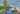Object detection and semantic segmentation

and that would work just fine, but what do you do when you have a lot more people such as Figure 1? Will the same assumptions hold true? Do we have access to the labelled datasets that are in the format used by R-CNN and its variants?

In this post, we are going to build models that attempt solve this using the pre-trained ConvNet as backbone and a regression head for counting the crowd.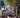Figure 1, source: ShanghaiTech Dataset

The network architecture is simple enough that I think this could be called the "Hello World" of the crowd density estimation task (pardon my ignorance if you know of simpler ways).High Level Flow

If we overlay the output, i.e., the density map over the image, we can see that the head of each person is highlighted. These highlighted points are what we want our model to learn to estimate. And, to get the total count, we sum the points together.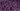Sample image and density map from ShanghaiTech Dataset

### Dataset

We use the crowd counting dataset introduced in this paper. The dataset is known as "ShanghaiTech Crowd Counting Dataset", and it has images with arbitrary crowd density along with the target labels. We train our model on Part A of the dataset. However, instead of using density maps provided by the dataset, we use the processed maps generated by the C3 Framework for convenience purposes. The C3 Framework is an excellent resource that covers multiple network architectures and their performance on different datasets. I encourage you to have a look at the paper and their repo.

Set
Total Images
Min People
Max People
Min Image Size
Max Image Size
Train300333136(400,400)(768,1024)
Test30012577(299,299)(768,768)

Histogram of train set

As you can see from the histogram, a large number of images have a crowd of less than 600.

Question 🤔: Does that mean the model trained on this dataset will not perform as well on the images with a large crowd?
Answer: You can use this notebook to ask this and other questions.

Below we show a few sample images from the dataset. We also show the associated density map below each image. Annotating the dataset must've been a difficult task.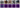Dataset sample

### Pre-processing

Throughout the implementation, we follow the guideline and techniques used by C3 Framework. The C3 framework uses the following augmentation/transformation:

CenterCrop (to 224) → RandomFlip → ScaleDown → LabelNormalize (100) → ToTensor → Normalize

1
2
3
4
5
6
7
8
9
10
11
12

# transforms
transforms.Compose([
CenterCrop(output_size=output_size),
RandomFlip(),
ScaleDown(factor), # Not used (currently set as 1)
LabelNormalize(),
ToTensor(),
Normalize()
])



Image transformations in Pytorch (syntax highlighter doesn't work yet😅)

## Models

We will use VGG16 as the backbone for our models in this post. Once we have the full training and evaluation infra ready, we can easily add more powerful models and compare its performance against the baseline models.

### Baseline Model

As our baseline, we will use a pre-trained VGG16 network followed by 2 Conv layers and the upsampling layer to match the target density map (m x n). Recollect that we scale down the input image and target by a scaling factor, so the final layer needs to keep that in consideration.VGG16 Baseline Model

1
2
3
4
5
6
7
8
9
10
11
12
13
14
15
16
17
18
19
20
21
22
23
24
25
26
27
28
29
30
31
32
33
34

class VGG16Baseline(nn.Module):
"""Baseline model

Baseline model that uses a pre-trained VGG16 network as backbone
"""
def __init__(self, channels=[512, 128, 1], scale_factor=4):
"""
Parameters
----------
channels: Input channel size for all three layers
scale_factor: Factor to upsample the feature map
"""
super(VGG16Baseline, self).__init__()
self.scale_factor = scale_factor
conv_layers = list(models.vgg16(pretrained=True).features.children())
# Mark the backbone as not trainable
for layer in conv_layers:

self.model = nn.Sequential(
*conv_layers,
nn.ReLU(inplace=True),
nn.ReLU(inplace=True)
)

def forward(self, inputs):
""" Forward pass"""
output = self.model(inputs)
output = F.upsample(output, scale_factor=self.scale_factor)
return output


Pytorch Code: models.py

We train the baseline model using the ADAM optimizer with the learning rate of 1e-5 for 400 epochs. The choice of loss function to optimizer is Mean Square Error(MSE). We also track Mean Absolute Error (MAE) on tensoboard along with the MSE.

Displaying only 200 epochs because the loss remained constant after that

#### Evaluation

We will first check how well the model is able to overfit the training data. We visualize its performance by comparing the actual vs. predicted crowd count. Each tab shows the predictions on the images of a given input size. The better the model, the more points lie closer to the diagonal line. As you can see in the plots below, the model does comparatively better for images with crowd ≤ 1000. However, its performance begins to suffer as the number of crowd increases.

Question for you: Recheck the histogram of training data and see if you can now guess the reason why?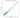Train set performance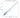Test set performance

I find it quite useful to review the images the model gets right and the ones where it struggles. Here, we display the images from both the train and test set.Train Set: Handpicked images from train set where the model does a good jobTrain Set: Images where the model does a poor job (marked in the red circle in the plot above)

Two things stand out to me: 1) the images with better prediction only contain people 2) the orientation of the heads in the image. Another crucial insight is that as you increase the input image size, the model starts to perform better. Review the plots in both 224x224 and 448x448 tabs to confirm this.

The sample from the test set also seems to confirm that the images with many different objects such as trees along with people make things difficult for the model. The last image is difficult for the human eye as well.Test Set: Images where the model does a poor job (marked in the red circle in test set performance plot)

### VGG16 with Decoder

We now move to another simple yet more powerful model that, too, uses pre-trained VGG16 as its backbone. We make use of CONV and CONVTRANSPOSE layers, the C3 paper refers to these layers as the decoder.VGG16 + Decoder Model

1
2
3
4
5
6
7
8
9
10
11
12
13
14
15
16
17
18
19
20
21
22
23
24
25
26
27
28
29
30
31
32
33
34
35

class VGG16WithDecoderV2(nn.Module):
""" VGG16 Decoder"""

def __init__(self):
super(VGG16WithDecoderV2, self).__init__()
conv_layers = list(models.vgg16(pretrained=True).features.children())[:23]
# Pre-trained layers are not trainable
for layer in conv_layers:

self.model = nn.Sequential(
*conv_layers,
nn.ReLU(inplace=True),
nn.ReLU(inplace=True),
nn.ReLU(inplace=True),
nn.ReLU(inplace=True),
nn.ReLU(inplace=True)
)

def forward(self, inputs):
"""forward pass

Parameters
----------
inputs: Batch of input images
"""
output = self.model(inputs)
return output


Pytorch Code: models.py

We train this model with the same hyper parameters as the baseline model for 400 epochs.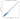Train set performance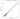Test set performance

According to the C3 Framework, both the models will have comparable performance but VGG16 with Decoder will generate more precise density maps. We can see this in the table and examples below. Our numbers are no way near the ones reported by C3 Framework, which I mainly think is because they use a higher input size to train their models.

Model
TRAIN: MSE
TEST: MSE
TRAIN: MAE
TEST: MAE
VGG16 Baseline144706613
VGG16 Decoder129650814

Next, we show a more detailed plot to visualise the effect of input image size on both the models.

MSE on different input size

And finally we overlay the density maps generated by both the models on a given image. We see that VGG16 Baseline is spot on ✅in terms of the actual count but VGG16 + Decoder generates tighter density maps.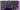VGG16 Baseline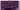VGG16 + Decoder

You can try increasing the input size and play around with the models yourself. If you can get good enough model, you can perhaps help answer the question on whose rally had more people🤣. The code is available on my GitHub Repo. In case you haven't noticed, if you hover over the cover image you will see the density map generated by VGG16 Decoder.

## What Next

You can try tuning the hyperparameters, finding the right learning rate and or model architecture to get better performance. Here is the list of things I would try next if I were to make it useful: